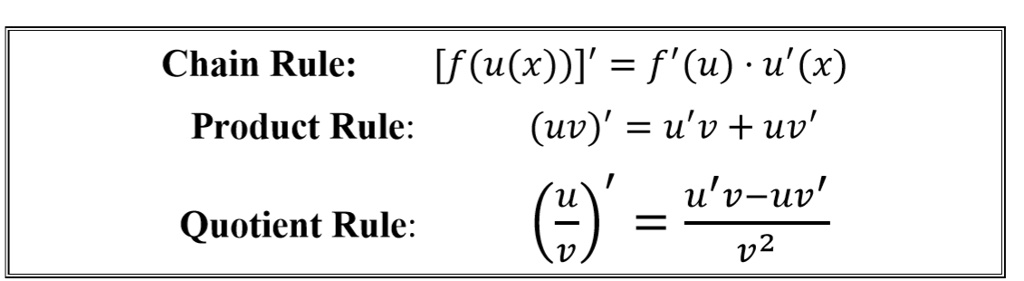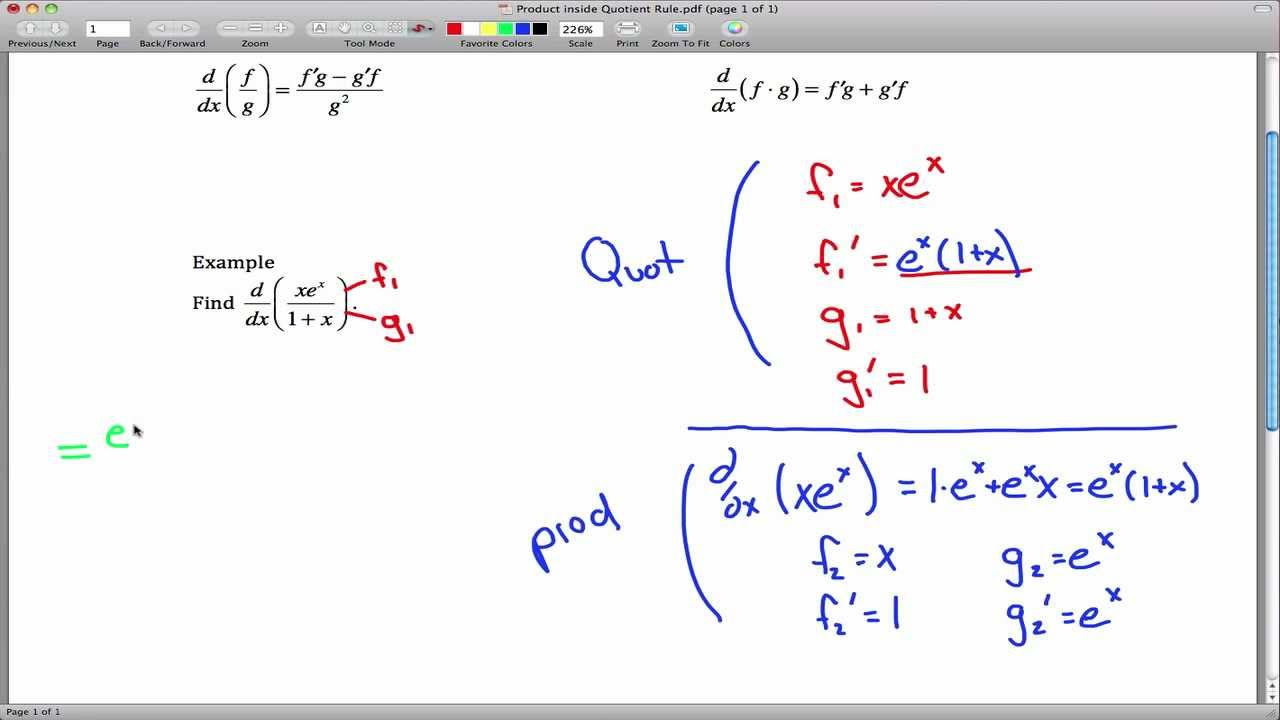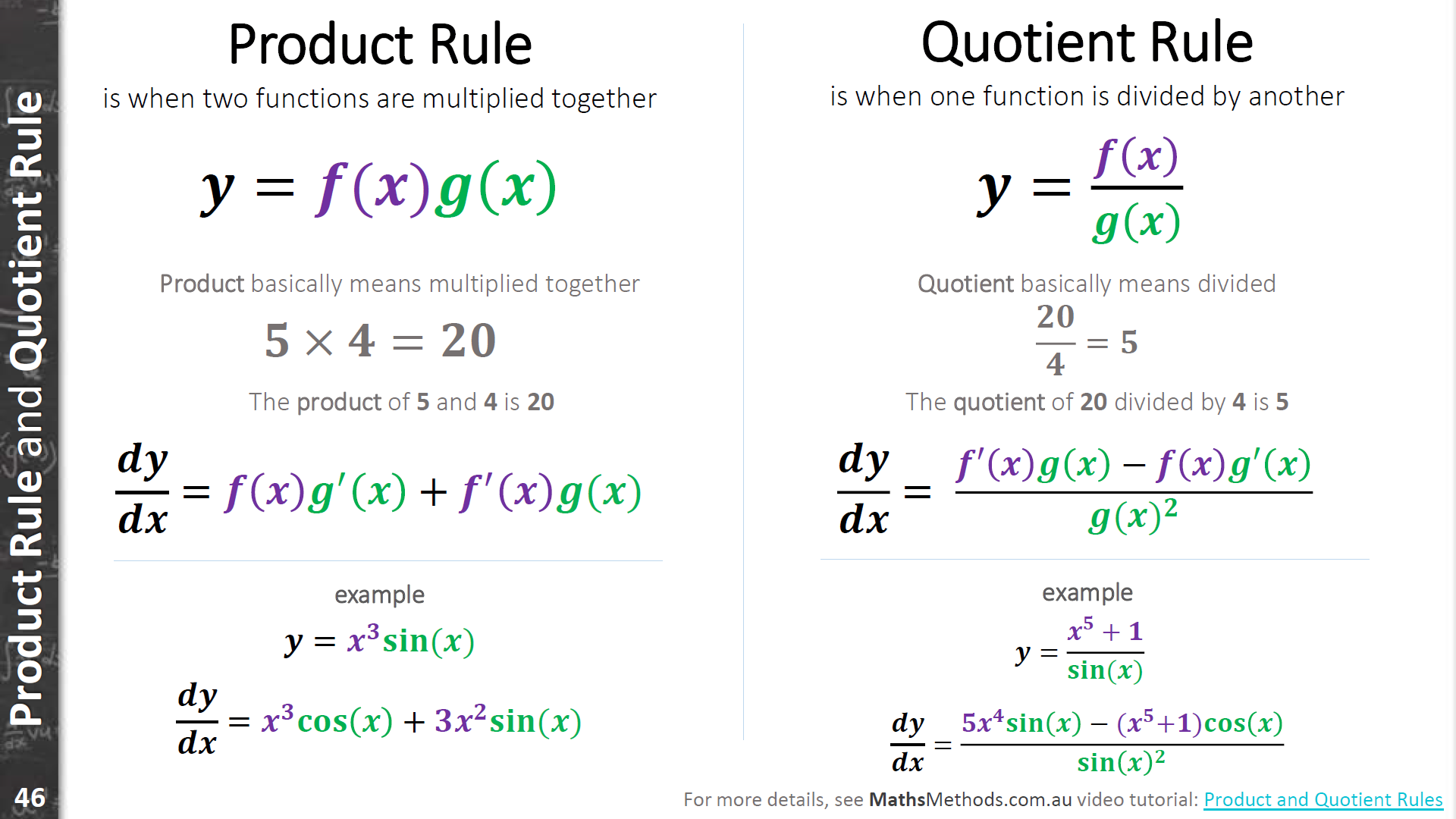Tuesday, 27 Sep 2022

# What is the quotient and product rule ?

So, if you are a high-school student pursuing science, calculus is almost like your bedmate. Math, physics, chemistry- name anything and you have it! However, Calculus is a tricky thing. So, you have lots of rules to remember if you want to do a sum in the best way possible. Therefore, the quotient and product rule is one of the most basic ones of them. These are some of the most elementary laws that you need for doing differentiation in calculus. Moreover, the quotient and product rule might act individually or in combination. However, we will see all that later. Now, let us look at them individually.So, in the quotient rule, one function divides another. However, there is a particular method that you have to apply here. Hence, start with the bottom function and end by squaring it. You can tell the quotient rule to be the product of the denominator and the derivative of the numerator subtracted from the product of the numerator and the derivative of the denominator. However, remember that throughout the calculation, the denominator is the square of the original denominator. It is not half as complex as it sounds. Don’t worry, you will get the explanation.

So again, the product rule is a staple for differentiation in calculus problems. In this case, you need to add the product of the first function’s derivative with the second function and the product of the second function’s derivative with the first function. So, this gives the product of the two functions’ derivatives. Hence, you should use the product rule when you are considering the quotient of two functions.

## Quotient and product rule derivatives

So, as we have already probably understood, there is no single quotient and product rule. Therefore, the quotient and product rule are really two different rules- the quotient rule and the product rule. So, when we will look at the derivatives of them, we have to handle them individually and not together as the quotient and product rule. Hence, let us take a look at them.

## Quotient rule derivative

Therefore, while using the quotient rule what we need to do is to find the derivative of the quotient of two functions.

So, let us say, your function in the numerator is f(x). On the other hand, in the denominator, you have the function g(x). So, the formula of the quotient rule derivative from this function would be-

d/dx (f(x)/g(x)) = ((g(x)* (d/dx)f(x))- (f(x) * (d/dx)g(x))/ ((g(x))^2).

So, here d/dx refers to the derivative of that term that we have attached to it. Hence, in this case, d/dx(f(x)) means the derivative of the numerator function f(x). On the other hand, d/dx(g(x)) means the derivative of the denominator function. Therefore, summing up, d/dx (f(x)/g(x)) refers to the derivative of the quotient of the two functions. So, we got the quotient part of the quotient and product rule. Now, let us take a look at the other one.## Product rule derivative

So, when do we use the product part of the quotient and product rule? We apply this one when we need to find the derivative of the product of two functions. Therefore, this one is a lot more simple than the quotient rule because here both the functions enjoy equal status. So, you can interchange them while calculating and you will still get the same derivative. We will see how.

So, suppose one of your functions is f(x). On the other hand, the other function that you have is g(x). Hence, the formula of the product rule derivative would be-

d/dx(f(x) * g(x)) = ( f(x) * d/dx(g(x))) + ( g(x) * d/dx(f(x))).

Therefore, here d/dx refers to the derivative of that term that we have attached to it. So, in this case, d/dx(f(x)) is the derivative of the function f(x). Similarly, d/dx(g(x)) means the derivative of the function g(x). Therefore, summing up, d/dx(f(x) * g(x)) refers to the derivative of the product of the two original functions. However, since it is pure multiplication and addition, it does not have the rigid process that the quotient part of the quotient and rule has. There if you swap terms, both meaning and answer will completely change.## Chain, product, and quotient rule

So, the name chain rule itself might suggest that there is a chain. The quotient and product rule somehow gets involved in the process. Moreover, the quotient and product rule is what forms the chain rule. Let us see what that means. So, it is often a matter of confusion as to where we should use the chain rule, where we must apply the product rule, and where the quotient rule. Moreover, there are high chances if you are a beginner that you might end up confusing yourself between the chain rule and the product part of the quotient and product rule because they are apparently very similar.## Difference between Chain rule and product rule

Therefore, when should we use the chain rule? We use it when we need to differentiate the function of a function. So, you understand that the terms of the bigger function here are themselves functions. And, when you do differentiation, you have to make sure, everything gets reduced to the smallest term. So, this comes in the format of d/dx ((f(g(x))). Hence, here x is a function of g(x). However, g(x) is again a function of f(g(x)). So you realize that there is a chain of functions. However, it is important to note that there is no multiplication between them. On the other hand, the product rule finds use when you just need to multiply two or more functions and then get the final term’s derivative. So, that comes in the format of d/dx ((f(x)) * (g(x))).

However, you have to understand that as the difficulty level of the problems increases, you have to use chain rule with quotient and product rule. Let us say you have to find the quotient of two functions. However, the terms of the numerator are themselves functions. So, there is a chain of functions. Hence, in this case, you have to use the quotient part of the quotient and product

rule at first. However, when you differentiate the contents inside while applying the quotient rule, you will need the chain rule as well. Therefore, you realize that the workings of these three are quite dependent on one another.

## Quotient and product rule combined

So, one thing that we must realize is that calculus is hardly simple. It is nearly impossible to do it with just a few basic rules. Therefore, we need a combination of many rules to get what we want. Think a little differently. So, we do not use calculus just for the sake of solving sums. There is a bigger purpose. So, intricate calculations in Physics or Chemistry where the scientists have to deal with minuscule quantities, calculus becomes extremely important.

Therefore, in such cases, you can hardly expect calculations to be so linear that you can just use one formula or a single rule and get the resultant value. Hence, as and when the sums become more difficult, they become more complex and you need to learn more and more techniques.

Hence, in most of the cases when you have learned it a bit, you would have to apply the quotient and product rule both for finding a single derivative. Moreover, it is also normal to use the chain rule in the process because the functions might contain many functions within them.

So, let us just consider a function like-

f(t) = 2t ( 4 (t-1) ^ 2 – 4t) / t (t – 1),

Therefore, here, you will have to use the chain rule, quotient rule as well as product rule to find out the derivative properly.## Quotient and product rule examples

So, there are sums that use the quotient and product rule individually. On the other hand, some use the quotient and product rule in combination. We will see examples of all the cases.

## Product rule example

Quo. f(t) = ( t – 2)(4t^2)

Ans. So, following the product rule,

d/df(t) = d/dt(t – 2) * 4 (t^2) + d/dt ( (4t^2) * (t – 2)

Now, d/dt (t – 2) gives 1 as 2 is constant which yields a 0 on differentiation. On the other hand, d/dt( 4 (t^2) gives 8t as per the power rules of differentiation.

Therefore, d/df(t) = 4(t^2) *1 + 8t (t – 2)

= 8t^2 + 4t^2 – 16t

= 12t^2 -16t.

= 4t (3t – 4).

## Quotient rule example

Quo. f(t) = 2t^2 / ( 4t -1)

Ans. So, following the quotient rule,

d/df(t) = ((4t -1)* (d/dt) 2t^2) – ( 2t^2 * (d/dt)( 4t -1)/ ((4t -1)^2)

Now,

d/dt of 2t^2 yields 4t as per power rules of differentiation. On the other hand, d/dt of 4t – 1 yields 4 because 1 is a constant which yields a zero on differentiation,

Therefore, d/df(t) = (( 4t( 4 t – 1) – (2t^2 * 4))/ (4t -1)^2

= ((16t^2 – 4t) – (8t^2))/ (4t -1)^2

= (8t^2 – 4t)/ (4t -1)^2

= 4t ( 2t -1)/ (4t -1)^2

## Quotient and product rule combined examples

So, this is the kind of sum that will face the most where you have to use the quotient and product rule in combination. Let us take a very quick look.

## 1.   f(t) = 2t ( 4 (t -1) ^ 2 – 4t) / t (t – 1)

So, as you can already see where you have to apply the quotient rule at first. However, on a closer look, you will realize that both the denominator and the numerator contain more than one term in multiplication. Therefore, you will need the product part of the quotient and product rule as well. So, you can apply both the quotient and product rule in combination simultaneously. This means while applying the quotient rule on a whole you are also applying the denominator and numerator individually. However, such a process might become very confusing and complicated after a few steps. So, let us take an alternative path.

Let us consider the numerator to be a and the denominator to be b. So, we will differentiate them separately at first using the product rule.

Therefore, a = 2t ( 4 (t -1) ^ 2 – 4t)

So, the terms here are 2t and (4 (t – 1)^2 – 4t)

Hence, following the product rule,

da/ dt = d/dt(2t) * (4 (t – 1)^2 – 4t) + d/dt (4 (t – 1)^2 – 4t) * 2t

Or, da/ dt = 2 (4 (t^2 – 2t + 1) – 4t) + (8 (t – 1) – 4) * 2t

So, da/ dt = 8 ( t^2 – 2t + 1) – 8t + ( 8t – 8 + 4) * 2t

Or, da/ dt = 8t^2 – 16t + 8 – 8t + 16t^2 – 16t + 8t

So, da/ dt = 24t^2 – 32t + 8

Now, b = t (t – 1)

So, the terms here are t and t – 1.

Hence, following the product rule,

db/ dt = d/dt (t) * (t – 1) + d/dt (t – 1) * t

Or, db/ dt = t – 1 + t

Or, db/ dt = 2t – 1.

Now, d(f(t))/dt = (b(da/ dt) – a(db/dt)) / b^2

Therefore, d(f(t))/dt = ((24t^2 – 32t + 8)(t^2 – 1) – ((2t – 1)(2t ( 4 (t -1) ^ 2 – 4t)) / (2t – 1)^2

## Quotient and product rule FAQs

### How do you know when to use the quotient and product rule?

Ans. So, if you want to find the derivative of the product of two functions, you have to use the product rule. On the other hand, if you want to find the derivative of the quotient of two functions, you have to use the quotient rule.### Can I use product rule instead of quotient?

Ans. Well, you should not ideally. Each has a different use, use that one accordingly. However, to avoid using the quotient rule in complicated situations, you can use the product rule cleverly. Check the examples for more references.### Does the quotient rule come from the product rule?

Ans. Yes, you can derive the quotient rule from a combination of the product rule and the chain rule.### Does the quotient rule order matter?

Ans. Yes, unlike in the product rule, the order in the quotient rule matters. So, this is because there is a subtraction involved. If you change the order, the value changes entirely.